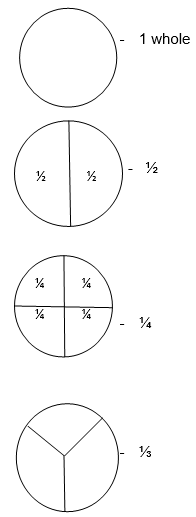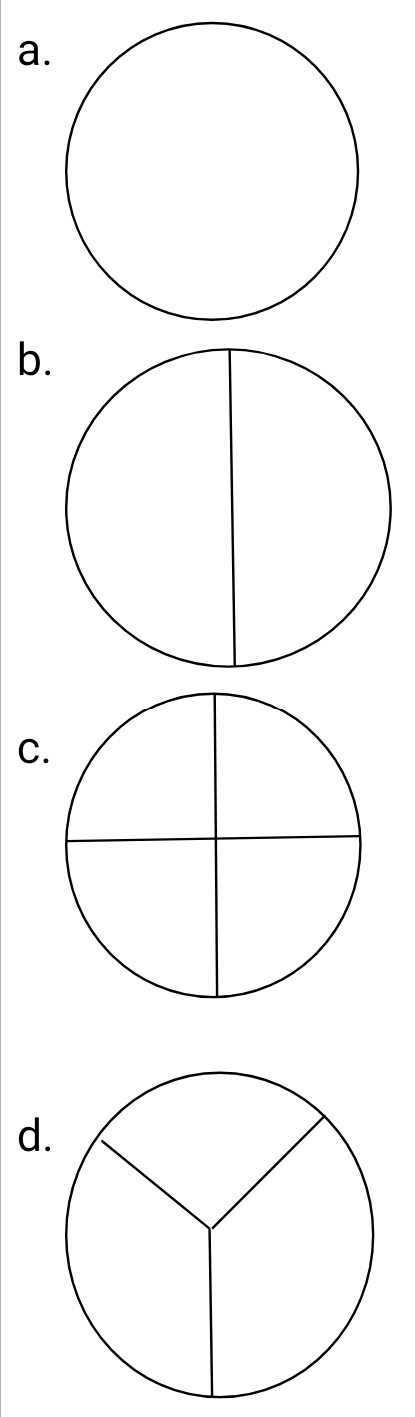# Lesson Notes By Weeks and Term - Nursery 2

Counting/identification of numbers 1 - 700

SUBJECT: Numeracy

TERM: 3rd Term

Week: 4

CLASS – Nursery 2

AGE – 5 years

DURATION – 5 periods of 35 minutes each

TOPIC- Counting/identification of numbers 1-700

CONTENT – Writing 400-450, Number endings. Number spellings 1-90. 10x table. Introduction to fractions, Telling time (o’clock). Sensorial-arranging like colours using bolts and nuts

INSTRUCTIONAL OBJECTIVES – By the end of the lesson, the pupils should be able to –

1. Count and identify numbers 1-700
2. Write numbers 400-450
3. Write in words 1-90
4. Recite the 10x table
5. Identify fractions
6. Tell the time
7. Arrange bolts and nuts based on their colours

INSTRUCTIONAL MATERIALS – flash cards, charts, counters

INSTRUCTIONAL TECHNIQUES – Explanation, repetition, questions and answers

ENTRY BEHAVIOUR – The pupils can count numbers 1-700 and spell numbers 1-80

SET INDUCTION –She uses number rhyme to arouse their interest

INSTRUCTIONAL PROCEDURE

PERIOD I: Counting numbers 1-700 and writing 400-450

 STEPS TEACHER’S ACTIVITY PUPILS’ ACTIVITY IIntroduction The teacher reviews the previous lesson introduces the new topic Pupils participate IICounting Using the number chart, she counts number 1-700 repeatedly. Pupils repeat after her IIIWriting numbers She counts and write numbers 400-450 on the board; she also uses flashcards Pupils count and write in their book IVNumber ending She writes the number endings on the board and also uses flashcards Pupils count Evaluation Count and write numbers 400-450 Conclusion She marks their books and corrects them where necessary

PERIOD II& III: Number spellings 1-90. Introduction to fractions, 10x times table

 STEPS TEACHER’S ACTIVITY PUPILS’ ACTIVITY IIntroduction The teacher reviews the previous lesson introduces the new topic Pupils participate IIDrilling She drills them on the spelling of 81-90Eighty-one = 81Eighty-two = 82Eighty-three = 83Eighty-four = 84Eighty-five = 85Eighty-six = 86Eighty-seven = 87Eighty-eight = 88Eighty-nine = 89Ninety = 90 IIIIntroduction to fractions She explains that fractions are a part of a wholeFor example;Pupils identify heavy and light objects III10x table She writes the 10x table on the board and recites it severally as the pupils recite after her10 x 1 = 1010 x 2 = 2010 x 3 = 3010 x 4 = 4010 x 5 = 5010 x 6 = 6010 x 7 = 7010 x 8 = 8010 x 9 = 9010 x 10 = 10010 x 11 = 11010 x 12 = 120 Evaluation 1. Identify these fractions2. Write in words 81-9081 =82 =83 =84 =85 =86 =87 =88 =89 =90 =3. Complete the 5x table10 x 1 =10 x 2 =10 x 3=10 x 4= Conclusion She marks their books and corrects them where necessary

PERIOD V: Telling time O’ clock. Sensorial- arranging bolts and nuts according to their colours

 STEPS TEACHER’S ACTIVITY PUPILS’ ACTIVITY IIntroduction The teacher reviews the basic colours and introduces the new topic Pupils respond IISorting bolts and nuts according to colours The teacher divides the pupils into four groups. Each group is to pick out the red, yellow, blue and green respectively Pupils sort out the bolts and nuts into colours IIITelling the time The teacher draws a clock face on the board and explains that O’ clock is simply when the minute hand is on 12 and the hour hand is on any other number Evaluation 1. The pupils are evaluated based on how well each group sorted out the bolts and nuts into colours2. The pupils are given exercises on O’ clock from their textbooks Conclusion She marks their books and corrects them where necessary